Question

# When a 23.8 mL sample of a 0.443 M aqueous hydrofluorie acid solution is titrated with...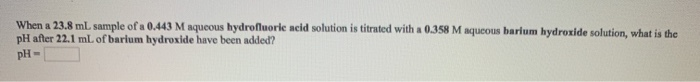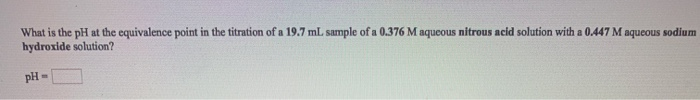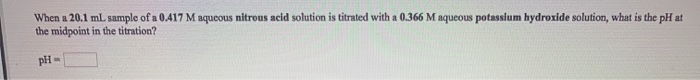When a 23.8 mL sample of a 0.443 M aqueous hydrofluorie acid solution is titrated with a 0.358 M aqueous barium hydroxide solution, what is the pH after 22.1 mL of barium hydroxide have been added? pH-
What is the pH at the equivalence point in the titration of a 19.7 mL sample of a 0.376 M aqueous nitrous acid solution with a 0.447 M aqueous sodium hydroxide solution? pH-
When a 20.1 mL sample of a 0.417 M aqueous nitrous acid solution is titrated with a 0.366 M aqueous potassium hydroxide solution, what is the pH at the midpoint in the titration? pH-

as for HOMEWORKLIB RULES i can only do first question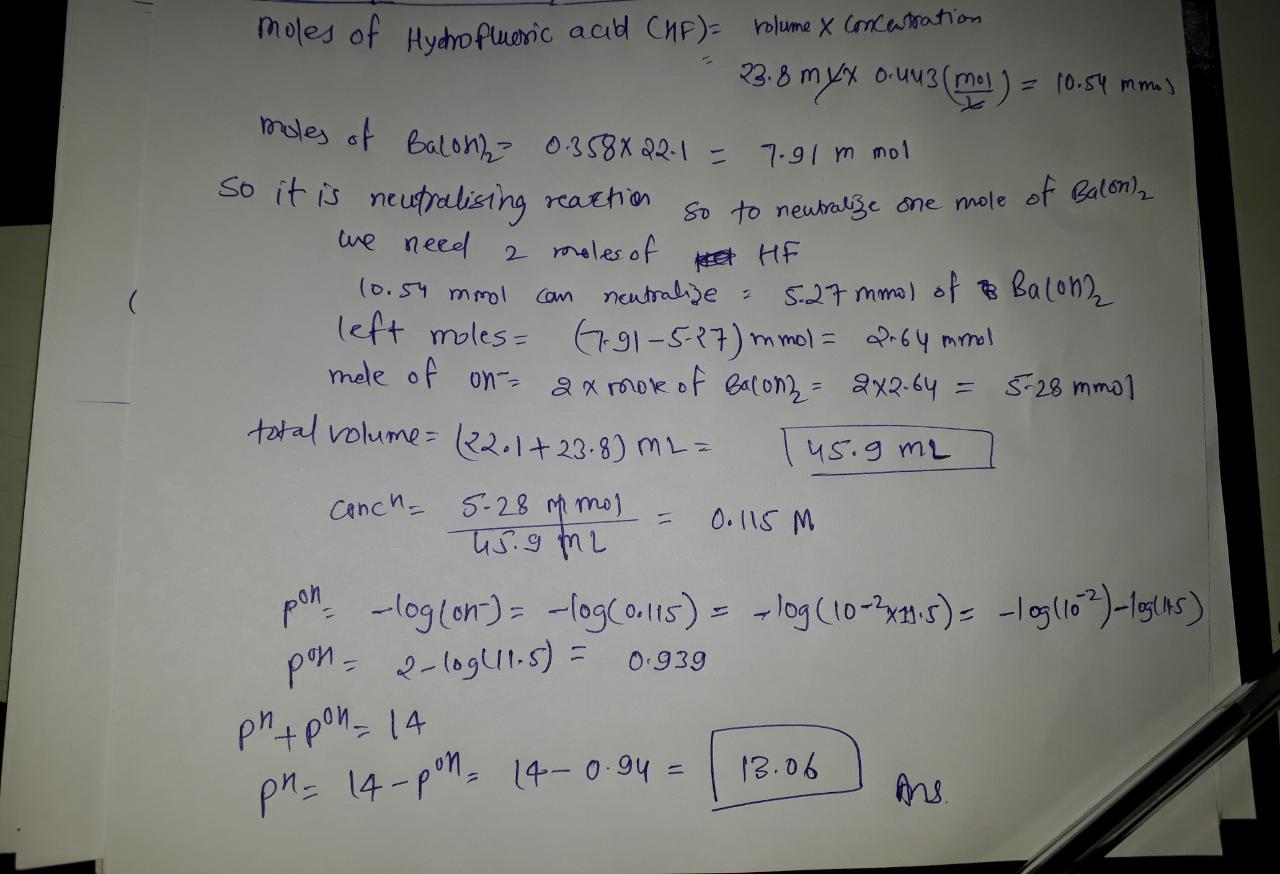#### Earn Coins

Coins can be redeemed for fabulous gifts.

Similar Homework Help Questions
• ### When a 23.8 mL sample of a 0.491 M aqueous acetic acid solution is titrated with...

When a 23.8 mL sample of a 0.491 M aqueous acetic acid solution is titrated with a 0.381 M aqueous potassium hydroxide solution, (1) What is the pH at the midpoint in the titration? (2) What is the pH at the equivalence point of the titration? (3) What is the pH after 46.0 mL of potassium hydroxide have been added

• ### When a 23.3 mL sample of a 0.443 M aqueous nitrous acid solution is titrated with...

When a 23.3 mL sample of a 0.443 M aqueous nitrous acid solution is titrated with a 0.305 M aqueous barium hydroxide solution, what is the pH after 25.4 mL of barium hydroxide have been added? pH =

• ### When a 24.9 mL sample of a 0.438 M aqueous acetic acid solution is titrated with...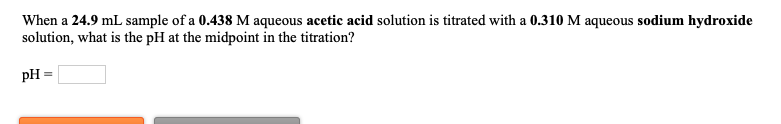When a 24.9 mL sample of a 0.438 M aqueous acetic acid solution is titrated with a 0.310 M aqueous sodium hydroxide solution, what is the pH at the midpoint in the titration? pH = What is the pH at the equivalence point in the titration of a 17.5 mL sample of a 0.380 M aqueous hydrofluoric acid solution with a 0.379 M aqueous barium hydroxide solution? PH = 3.14

• ### When a 17.2 mL sample of a 0.324 M aqueous hypochlorous acid solution is titrated with...

When a 17.2 mL sample of a 0.324 M aqueous hypochlorous acid solution is titrated with a 0.319 M aqueous barium hydroxide solution, (1) What is the pH at the midpoint in the titration? (2) What is the pH at the equivalence point of the titration? (3) What is the pH after 13.1 mL of barium hydroxide have been added?

• ### 1. When a 21.5 mL sample of a 0.329 M aqueous hypochlorous acid solution is titrated...

1. When a 21.5 mL sample of a 0.329 M aqueous hypochlorous acid solution is titrated with a 0.456 M aqueous potassium hydroxide solution, what is the pH after 23.3 mL of potassium hydroxide have been added? pH = 2. When a 16.0 mL sample of a 0.404 M aqueous hydrocyanic acid solution is titrated with a 0.418 M aqueous barium hydroxide solution, what is the pH at the midpoint in the titration? pH =

• ### When a 19.8 mL sample of a 0.460 M aqueous acetic acid solution is titrated with...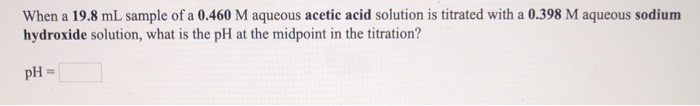When a 19.8 mL sample of a 0.460 M aqueous acetic acid solution is titrated with a 0.398 M aqueous sodium hydroxide solution, what is the pH at the midpoint in the titration? pH = A 17.5 mL sample of a 0.430 M aqueous hydrofluoric acid solution is titrated with a 0.381 M aqueous potassium hydroxide solution. What is the pH at the start of the titration, before any potassium hydroxide has been added? pH-

• ### When a 21.4 mL sample of a 0.311 M aqueous hydrocyanic acid solution is titrated with...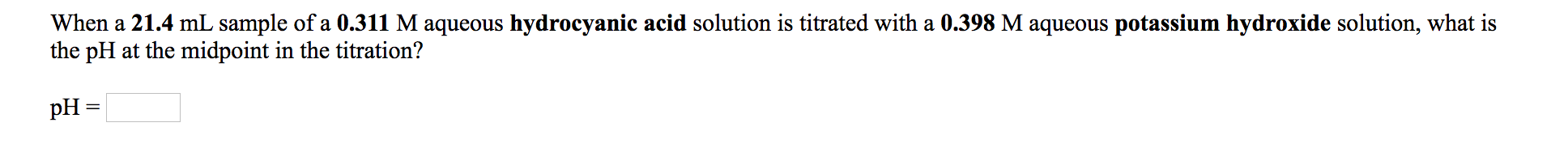When a 21.4 mL sample of a 0.311 M aqueous hydrocyanic acid solution is titrated with a 0.398 M aqueous potassium hydroxide solution, what is the pH at the midpoint in the titration? pH = A 45.3 mL sample of a 0.370 M aqueous acetic acid solution is titrated with a 0.424 M aqueous potassium hydroxide solution. What is the pH after 23.1 mL of base have been added? pH =

• ### 1) A 34.2 mL sample of a 0.590 M aqueous acetic acid solution is titrated with...

1) A 34.2 mL sample of a 0.590 M aqueous acetic acid solution is titrated with a 0.203 M aqueous potassium hydroxide solution. What is the pH after 37.1 mL of base have been added? 2) What is the pH at the equivalence point in the titration of a 25.5 mL sample of a 0.351 M aqueous hypochlorous acid solution with a 0.433 M aqueous barium hydroxide solution?

• ### A 23.0 mL sample of a 0.497 M aqueous acetic acid solution is titrated with a...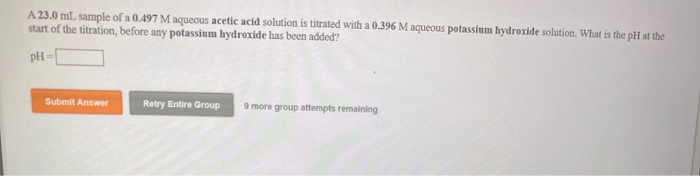A 23.0 mL sample of a 0.497 M aqueous acetic acid solution is titrated with a 0.396 M aqueous potassium hydroxide solution. What is the pH at the start of the titration, before any potassium hydroxide has been added? PH Submit Answer Retry Entire Group 9 more group attempts remaining What is the pH at the equivalence point in the titration of a 25.5 mL sample of a 0.411 M aqueous acetic acid solution with a 0.352 M aqueous barium...

• ### When a 23.7 mL sample of a 0.324 M aqueous hypochlorous acid solution is titrated with...

When a 23.7 mL sample of a 0.324 M aqueous hypochlorous acid solution is titrated with a 0.328 M aqueous barium hydroxide solution, what is the pH at the midpoint in the titration? pH =